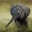Related Tags

c++
heapify
max-heap

# How to merge two binary max-heaps into a new max-heapChayshta Singh

### Merge binary max-heaps to create new max-heap

This Answer will explain how to merge two binary max-heaps given as arrays to create a new max-heap.

### Algorithm

The most straightforward solution is to first create a new array by copying the elements of both heaps. We then build a max-heap with the newly created array.

We use the max-heapify operation to build max-heaps.

1. maxHeapify(idx, heap) assumes that the root of the max-heap is at the index idx and both its children are already max-heaps. If the element at idx is not the maximum element, it swaps with the maximum of its left and right children, and then recursively calls itself on the affected child sub-heap until the max-heap property is restored.
2. We build a max-heap by iteratively calling maxHeapify(idx, heap). This follows a bottom-up approach by building sub-heaps starting at the last element with children and going backward to the root. If N-1 is the index of the last element in heap, then its parent's index is ((N-1) - 1)/2 i.e N/2 - 1. This is the last element with children. All childless nodes are already max-heaps.
int N = heap.size();
for(int i = N/2 - 1; i >= 0; i--)
maxHeapify(i, heap);

### Code example

#include <iostream>
#include <vector>
#include <algorithm>

//Merge two binary max-heaps given as arrays to form a new max-heap
void maxHeapify(int k, std::vector<int> & heap){
if(k >= heap.size())		//base condition
return;
int lc = 2 * k + 1;		//left child index
int rc = 2 * k + 2;		//right child index
int maxIndex = k;
// if left child is greater than parent(at k)
if(lc < heap.size() && heap[lc] > heap[maxIndex])
maxIndex = lc;
// if right child is greater than greatest (at maxIndex)
if(rc < heap.size() && heap[rc] > heap[maxIndex])
maxIndex = rc;

//if greatest is not parent k
if(maxIndex != k){
std::swap(heap[maxIndex], heap[k]);
//recursively heapify the affected sub-heap
maxHeapify(maxIndex, heap);
}
}

std::vector<int> mergeMaxHeaps(std::vector<int> const & arr1, std::vector<int> const & arr2){

std::vector<int> heap;
//append arr1 and arr2 to heap
std::copy(arr1.begin(), arr1.end(), std::back_inserter(heap));
std::copy(arr2.begin(), arr2.end(), std::back_inserter(heap));

//build a max-heap
int N = heap.size();
for(int i = N/2 - 1; i >= 0; i--){
maxHeapify(i, heap);
}
return heap;
}

void DrawMaxHeap(std::vector<int> const & h);

int main(){
std::vector<int> const maxHeap1{9, 4, 7, 1, -2}; //input max-heap 1
std::vector<int> const maxHeap2{8, 6 , 3, 5}; // input max-heap 2
std::cout << "Max-heap 1 : ";
DrawMaxHeap(maxHeap1);
std::cout << "Max-heap 2 : ";
DrawMaxHeap(maxHeap2);
auto res = mergeMaxHeaps(maxHeap1, maxHeap2);
std::cout << "Merged max-heap: ";
DrawMaxHeap(res);
return 0;
}

Merging two binary max-heaps

### Time complexity

The worst-case time complexity of the maxHeapify function is O(log N), where N is the number of elements in the max-heap. In this case, N is the sum of the sizes of the two input max-heaps.

While it appears that we perform N/2 * O(log N) operations to build the max-heap, the actual time complexity is O(N). This is because the number of swap operations is reduced when the children of each node are already max-heapified.

### Code explanation

Line 11–17: We find the index of the maximum element maxIndexof the max-heap with its root at index k of heap.

Line 20–24: If heap[k]is not the maximum element, we swap heap[k] and heap[maxIndex]. This might affect the heap property in the sub-heap with its root at maxIndex, so we recursively call maxHeapify(maxIndex, heap).

Line 31–32: We copy the elements of arr1 and arr2 in heap using copy function. std::back_inserter(heap) ensures that elements are inserted at the end of heap.

Line 36–38: We build the max-heap iteratively starting at the last element, with children at the index N/2 - 1 and going back to 0.

RELATED TAGS

c++
heapify
max-heap

CONTRIBUTORChayshta Singh
RELATED COURSES

View all Courses

Keep Exploring

Learn in-demand tech skills in half the time